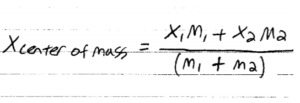Blog / Center Of Mass

### Center of Mass

Written by Chau Pham on Feb 26, 2020

The center of mass of an object is the point that moves as though all of the system’s mass were concentrated there and all external forces were applied there.

When solving physics problems, we tend to treat an object as a point. This is normally the point where you anchor your force vectors in free-body diagram. However, in reality objects have volume. In order to keep treating an object as a point and make it easy for us to apply the strategies so far, we can use the center-of-mass concept. This is a point where all the mass of the system sits.

Consider the situation where  two particles of masses m1 and m2 separated by distance d. We can find its center-of-mass by:If an object has a point, a line, or a plane of symmetry. The center of mass of such an object then lies at that point, on that line, or in that plane. For example, the center of mass of a uniform sphere is at the center of the sphere (which is the point of symmetry). The center of mass of a uniform cone (whose axis is a line of symmetry) lies on the axis of the cone. The center of mass of a banana (which has a plane of symmetry that splits it into two equal parts) lies somewhere in the plane of symmetry.

The center of mass of an object need not lie within the object. There is no dough at the com of a doughnut, and no iron at the com of a horseshoe.

KEY TAKEAWAYS

Key points:

• The Center of Mass (CoM) is a point as though all system’s mass were concentrated and all external forces were applied.
• The coordinate of the CoM can be found by taking integration of the particles along its 3-axes
• If an object is symmetrical along a point, line or plane, the CoM then lies at that point, on that line, or in that plane.

Key terms:

• Mass: physical property of matter that depends on size and shape of matter, and is expressed as kilograms by the SI system.
• Symmetry: Exact equivalence on either side of a dividing line, plane, center or axis.

Billing Information